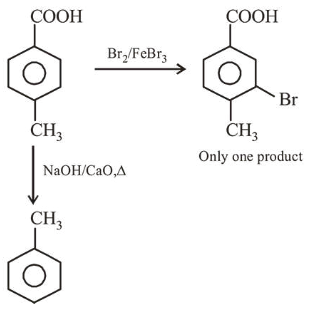# Solve the following

Question:

$[\mathrm{P}]$ on treatment with $\mathrm{Br}_{2} / \mathrm{FeBr}_{3}$ in $\mathrm{CCl}_{4}$ produced a single isomer $\mathrm{C}_{8} \mathrm{H}_{7} \mathrm{O}_{2} \mathrm{Br}$ while heating [ $\mathrm{P}$ ] with sodalime gave toluene. The compound [P] is :

1.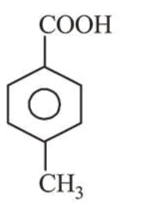2.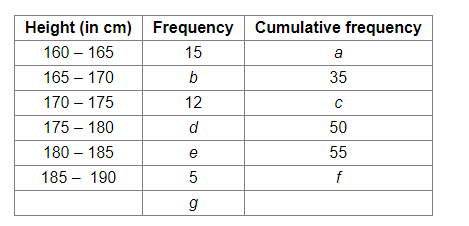3.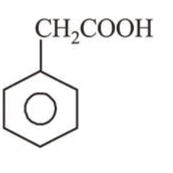4.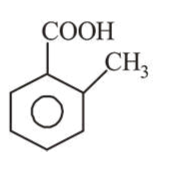Correct Option: 1

Solution: